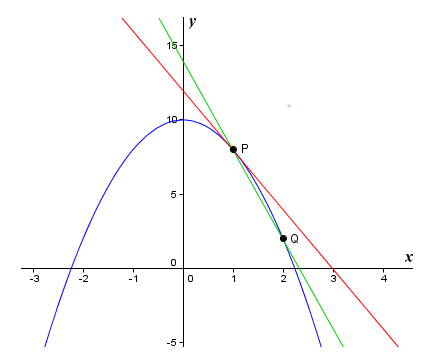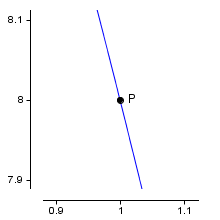# First Principles of Differential Calculus

Differentiation is about finding the instantaneous rate of change of a function. For a linear function this is a trivial exercise because the graph of the function is a straight line. If you look at the graph of ƒ(x) = x/2 (below), you can see that when x increases by two (2), y increases by one (1). The slope of the graph is therefore 1/2 for every point on the graph. The instantaneous rate of change of y with respect to x at any point on the graph of a function is equal to the slope of the graph at that point. For a linear function, the instantaneous rate of change will be the same for any value of x.The graph of the linear function ƒ(x) = x/2

When we start to look at non-linear functions, things get a little more complicated. Consider the graph of the function ƒ(x) = x3 - 9x - 14:The graph of the non-linear function ƒ(x) = x3 - 9x - 14

You can see that the slope of the graph varies continuously as the value of x changes. We have shown the tangent to the graph (the straight line drawn in red) at point P. The slope of the tangent gives us the slope of the graph at point P, and represents the instantaneous rate of change of y with respect to x - i.e. the derivative - at point P. The question is, how do we find the slope of the tangent (derivative) at some arbitrary point on a curve? Consider the graph of the non-linear function ƒ(x) = 10 - 2x2:The graph of the non-linear function ƒ(x) = 10 - 2x2

We want to find the slope of the tangent to point P (shown here in red). We have arbitrarily chosen a second point on the graph, which we will call point Q. The x coordinates for points P and Q are one (1) and two (2) respectively. The green line on the graph represents the secant that intersects points P and Q (a secant is a straight line that intersects a curve at two points). The slope of the secant approximates (is close to) that of the tangent. How can we get a better approximation? You would be correct if you said that we need to move Q closer to P. Let's see what happens when we move Q to the point on the graph where x is equal to one-point-five (1.5).The slope of the lines starts to converge as Q gets closer to P

As expected, the slope of secant PQ is now closer to that of the tangent. If we continue to move Q closer to P, the secant will eventually become virtually indistinguishable from the tangent. If we put Q close enough to P, we can get a good approximation of the slope of the tangent by finding the slope of the secant. Let's do that now. You may recall that in order to find the slope of a straight line, we just need to know the x and y coordinates of two points on the line. We already have the x coordinates for points P and Q. We can get the y coordinates by plugging these x coordinates into the function ƒ(x) = 10 - 2x2, as shown here:

yP  =  10 - 2x2  =  10 - 2  =  8

yQ  =  10 - 2x2  =  10 - 4.5  =  5.5

You may recall that if we have two points with xy coordinates x1, y1 and x2, y2, the general formula for finding the slope m of the straight line that intersects both points is:

 m  = y2  -  y1 x2  -  x1

Plugging the x and y coordinates of points P and Q into this formula we get:

 m  = 8  -  5.5 =  -5 1  -  1.5

We now have an approximation for the slope of the graph at point P. If we look at the graph, however, the secant is still noticeably steeper than the tangent, so our approximation is not particularly accurate. As we have already mentioned, we can approximate the tangent line more accurately by moving point Q close to point P. As the distance between the two points becomes smaller, our approximation of the slope of the tangent becomes more accurate. This means we should choose a value of x for Q that is very close to one (1). It is interesting to look at what happens to the slope of our secant as we choose x coordinates for Q that are progressively closer to one. We present the results below in tabular form.

xPyPxQyQSlope P-Q
1.0000008.0000001.1000007.580000-4.200000
1.0000008.0000001.0100007.959800-4.020000
1.0000008.0000001.0010007.995998-4.002000
1.0000008.0000001.0001007.999600-4.000200
1.0000008.0000001.0000107.999960-4.000020
1.0000008.0000001.0000017.999996-4.000002

From these results, we can see that the slope of the tangent is getting closer and closer to minus four (-4). We could reasonably conclude that, as the x coordinate of point Q approaches one, the slope of secant PQ will approach minus four. How can we express this formally? Consider the following expression:

 lim yQ - yP =  -4 xQ→1 xQ - xP

This is the standard formula for the slope of a straight line, with the x and y coordinates of points P and Q plugged into it. The notation on the left-hand side of the expression might look a little strange to you, though. We see the word lim above the expression xQ→1. The word lim tells us that we are approaching some limit (in this case the slope of the tangent to point P). The expression xQ→1 tells us that we are approaching this limit as the value of xQ (the x coordinate of point Q) approaches one. We use the idea of a limit to represent some value that we can approach, but never quite reach. At first, we don't know exactly what that value is, but as we get closer and closer to it, we can see what it's going to be.

Let's think about what we are doing here. We want to find the slope of the graph of a non-linear function ƒ for some point on the graph of the function. We already know the value of the x coordinate for that point. We'll just call it x. We don't really need to specify some arbitrary second point on the graph to be able to apply our slope formula. We just need to choose a suitably small value to represent the horizontal distance h between the two points on the graph. The value of h can be anything we choose - the smaller the better. The x coordinate of our second point can then be expressed simply as x + h. We have already seen that the y coordinates of these two points can be obtained by plugging the corresponding x coordinates into the non-linear function. We can therefore rewrite our formula for the slope of the tangent (i.e. the derivative) at some point P as follows:

 Slope of tangent to P  = lim = ƒ(x+h) - ƒ(x) h→0 h

We can make the value of h very small, but it can never be allowed to become zero since that would result in our formula having zero in the denominator. Consequently, the approximation we get for the derivative at point P approaches the true value as h approaches zero, but will never quite reach it. Nevertheless, by making h smaller and smaller we are effectively "zooming in" on point P. If we could zoom in indefinitely, we would eventually see a very short section of the graph centered on P that is, to all intents and purposes, a straight line. The illustration below shows what the graph of the function ƒ(x) = 10 - 2x2 looks like if we magnify the small section on which P lies.This small section of the graph is almost a straight line

One advantage of using h to represent the horizontal distance between point P and some arbitrary second point close to P on the graph is that we can use both positive and negative values for h. If we use values that are greater than zero (h>0), we will effectively be finding the limit of the slope as the second point approaches P from the right. If we use values that are less than zero (h<0), we will be finding the limit of the slope as the second point approaches P from the left. This can be useful if we need to differentiate a function that has some kind of discontinuity on one side of the point we want to differentiate. A function can only be said to be continuous if the graph of the function is a smooth curve, with no gaps or sudden breaks.